# Selina Solutions Concise Maths Class 7 Chapter 13: Set Concepts

Selina Solutions Concise Maths Class 7 Chapter 13 Set Concepts help students understand the fundamental concepts, which are important in higher classes also. We all know that a set is a collection of well defined objects, symbols or things, etc. In a subject like Mathematics, collection of objects is called a set. Important terms from the exam point of view are explained in brief in this chapter. For further details, students can avail Selina Solutions Concise Maths Class 7 Chapter 13 Set Concepts PDF, from the links which are available below.

Chapter 13 provides students with a clear idea of basic concept, elements, representation, types of set and operations. The solutions are provided both in exercise and chapter wise format, which can be used by students in both online and offline mode.

## Selina Solutions Concise Maths Class 7 Chapter 13: Set Concepts Download PDF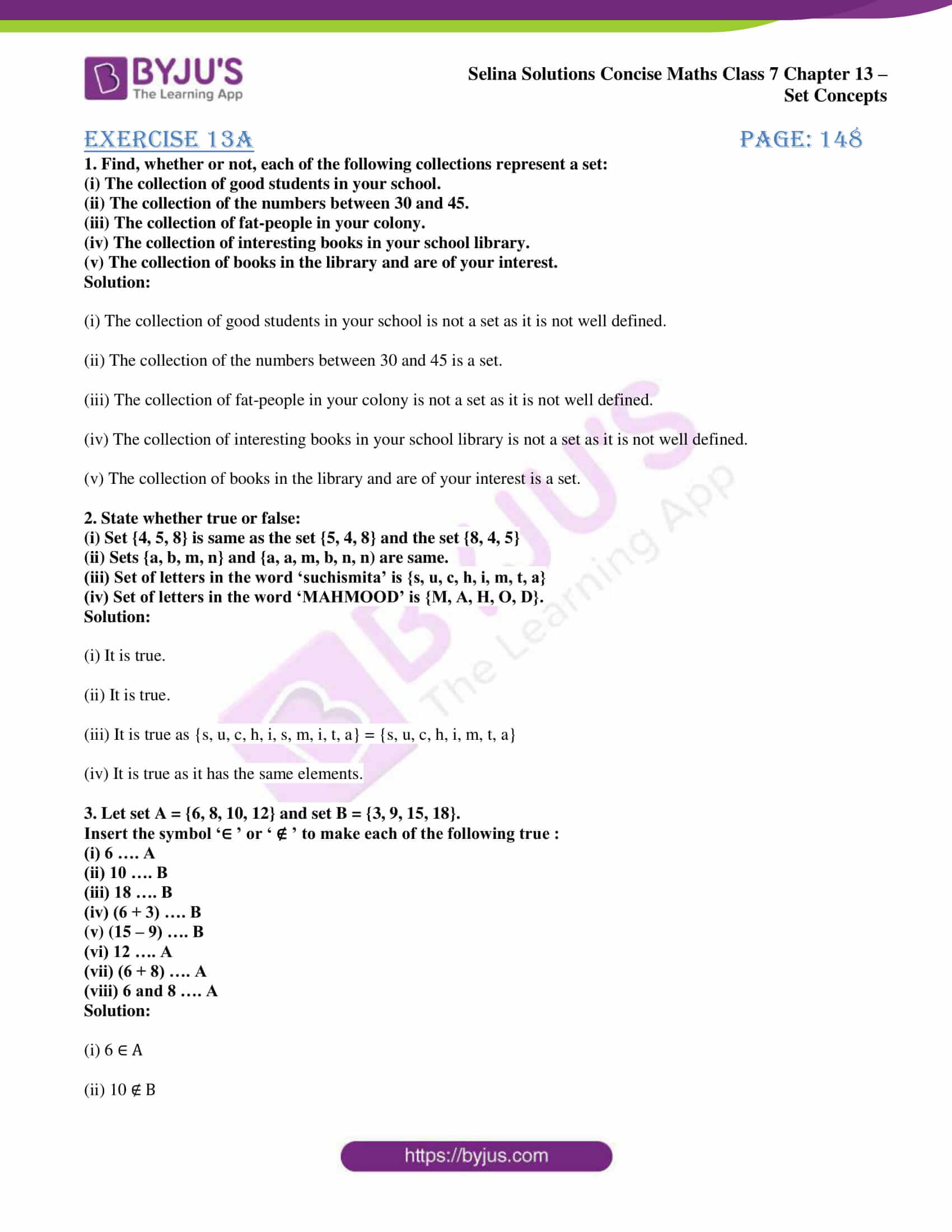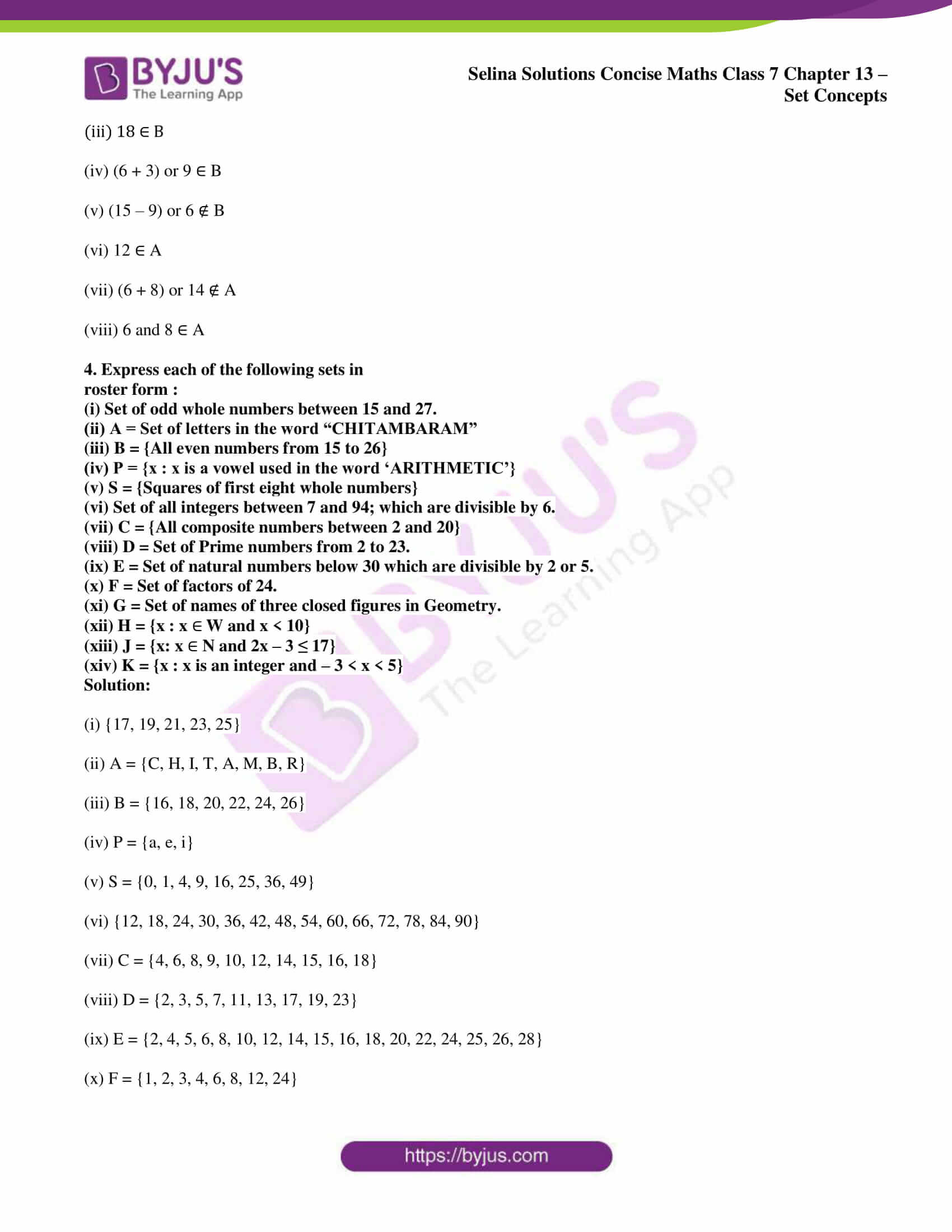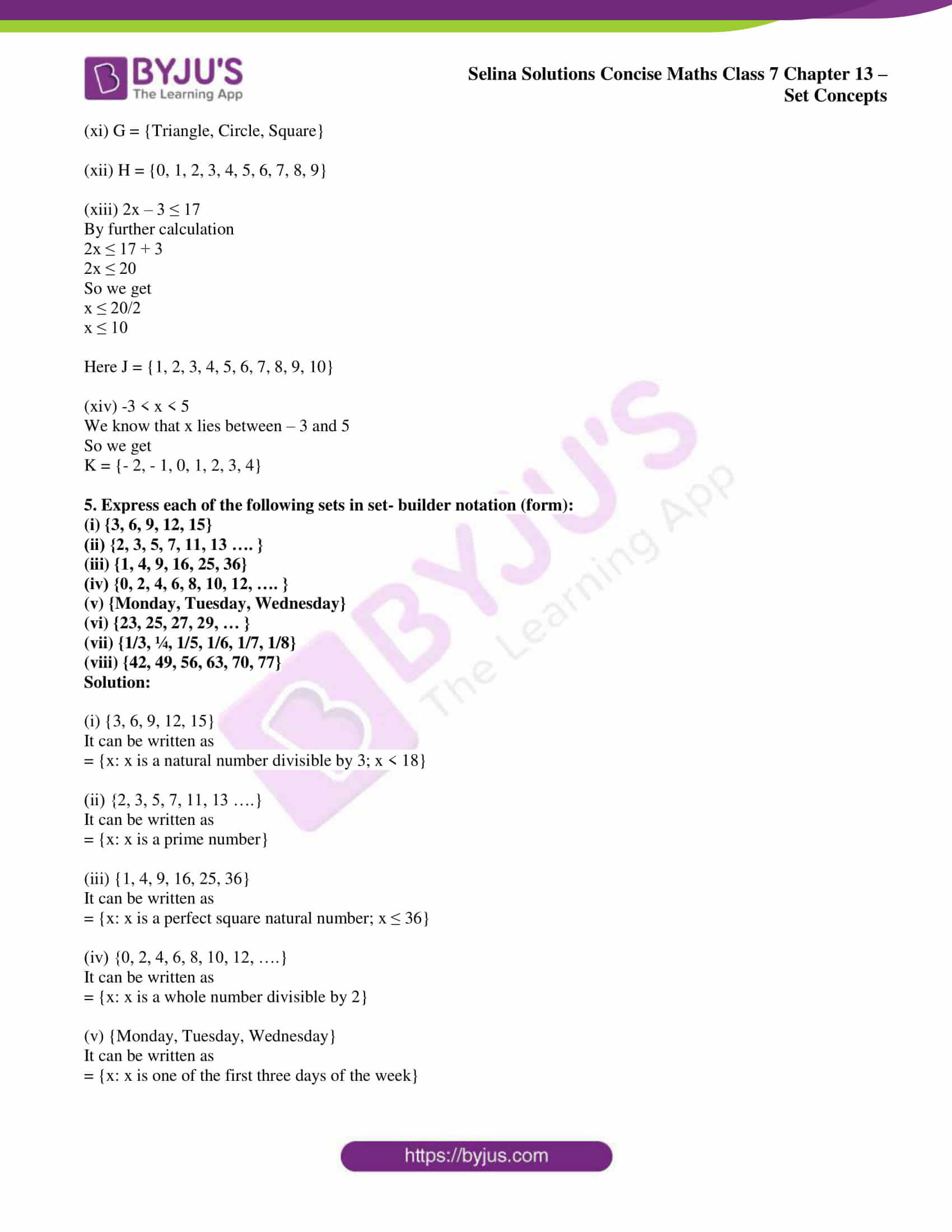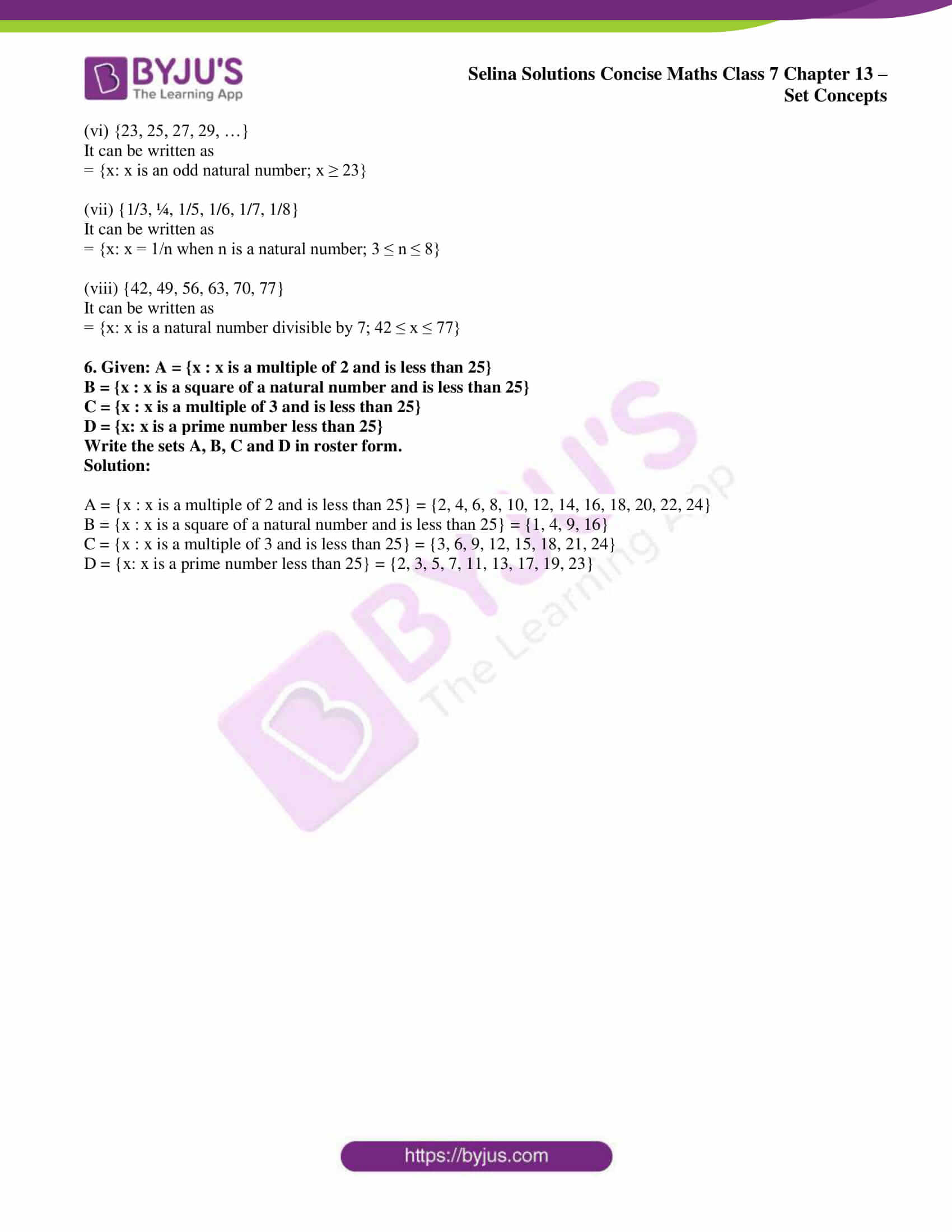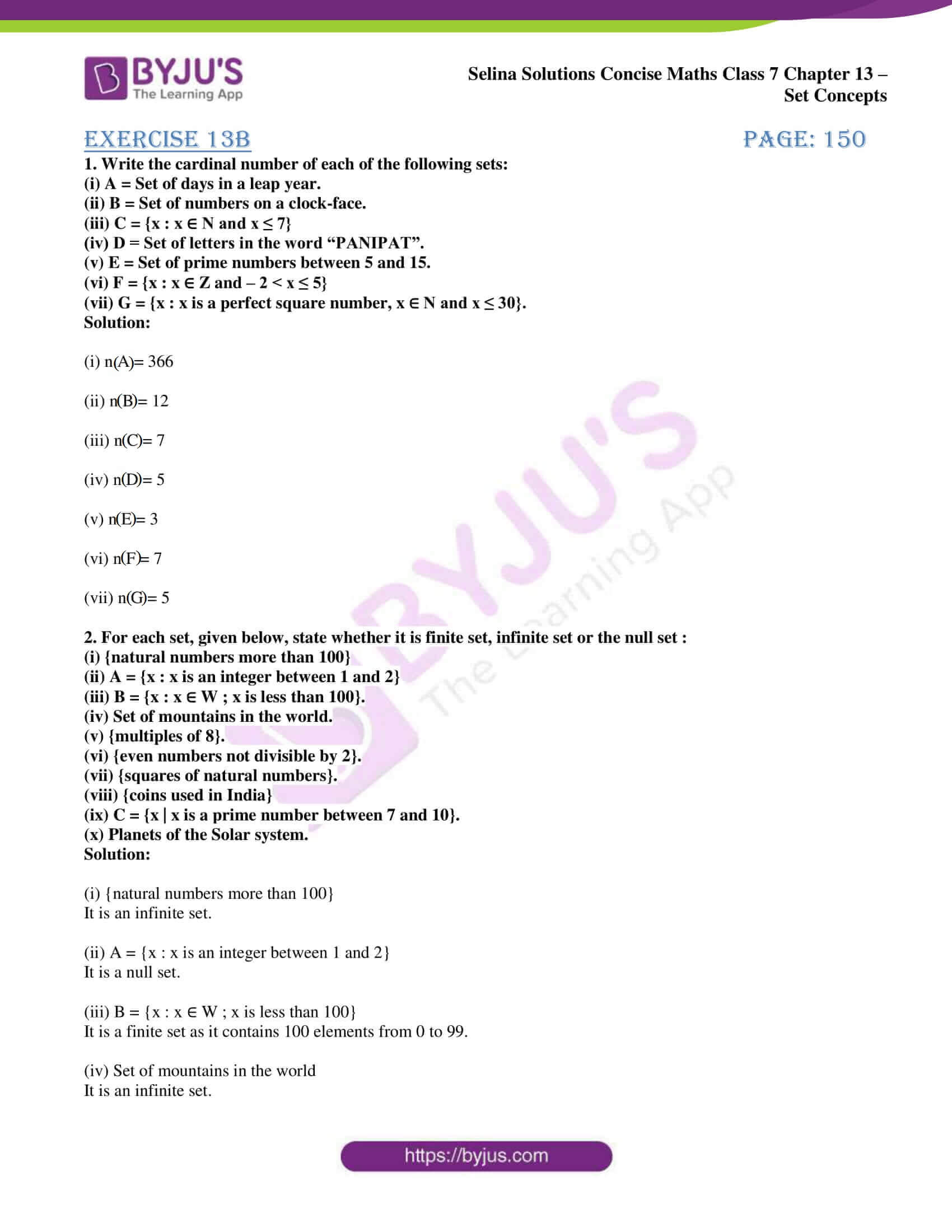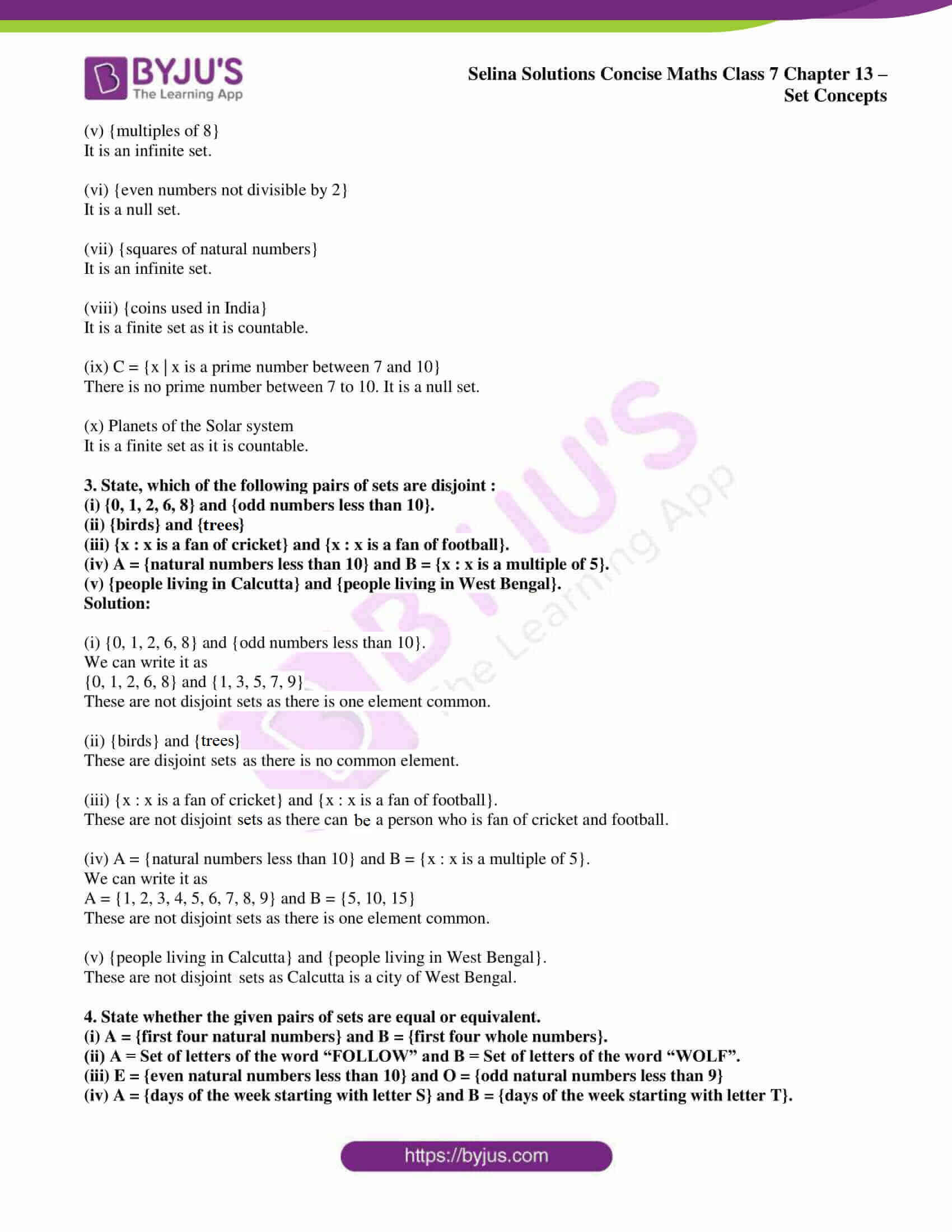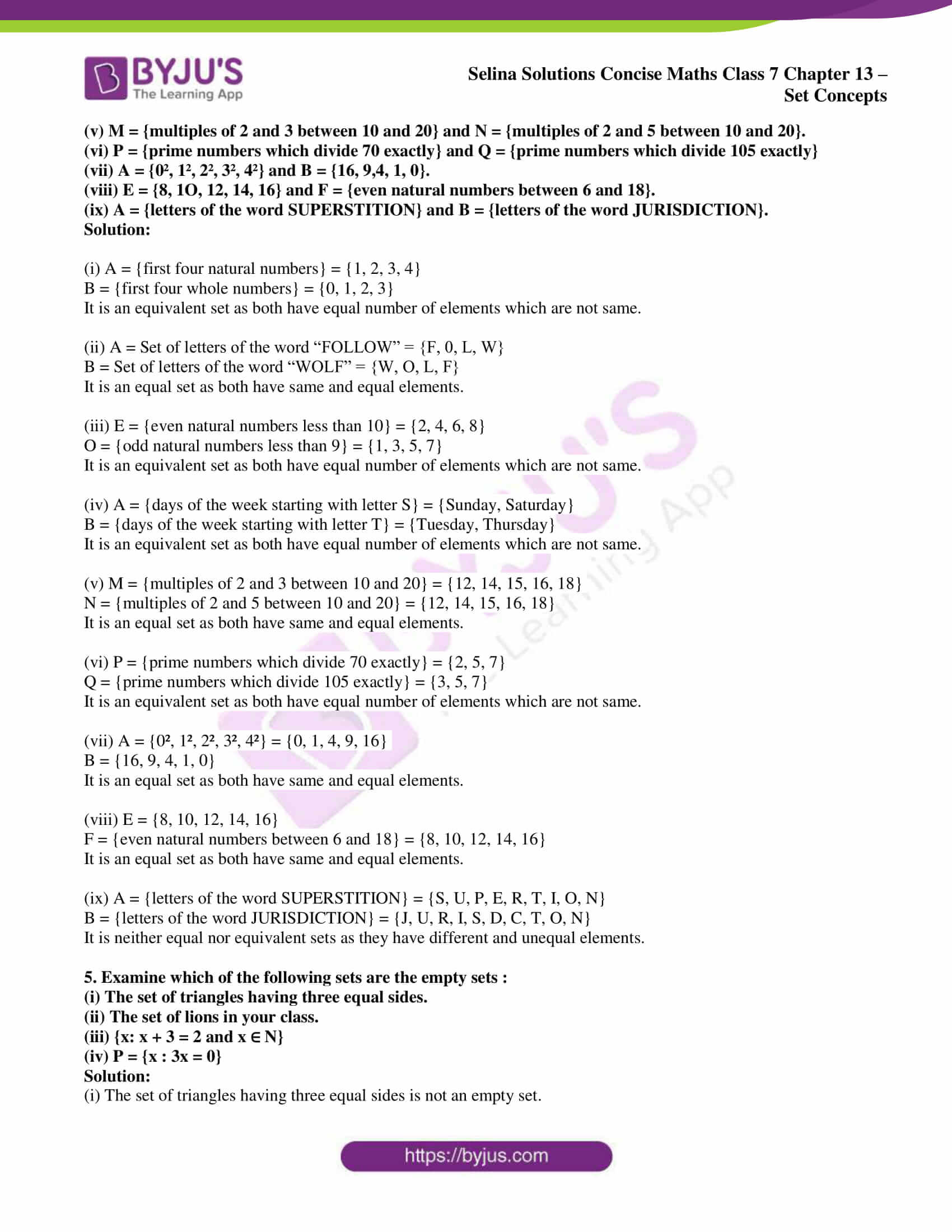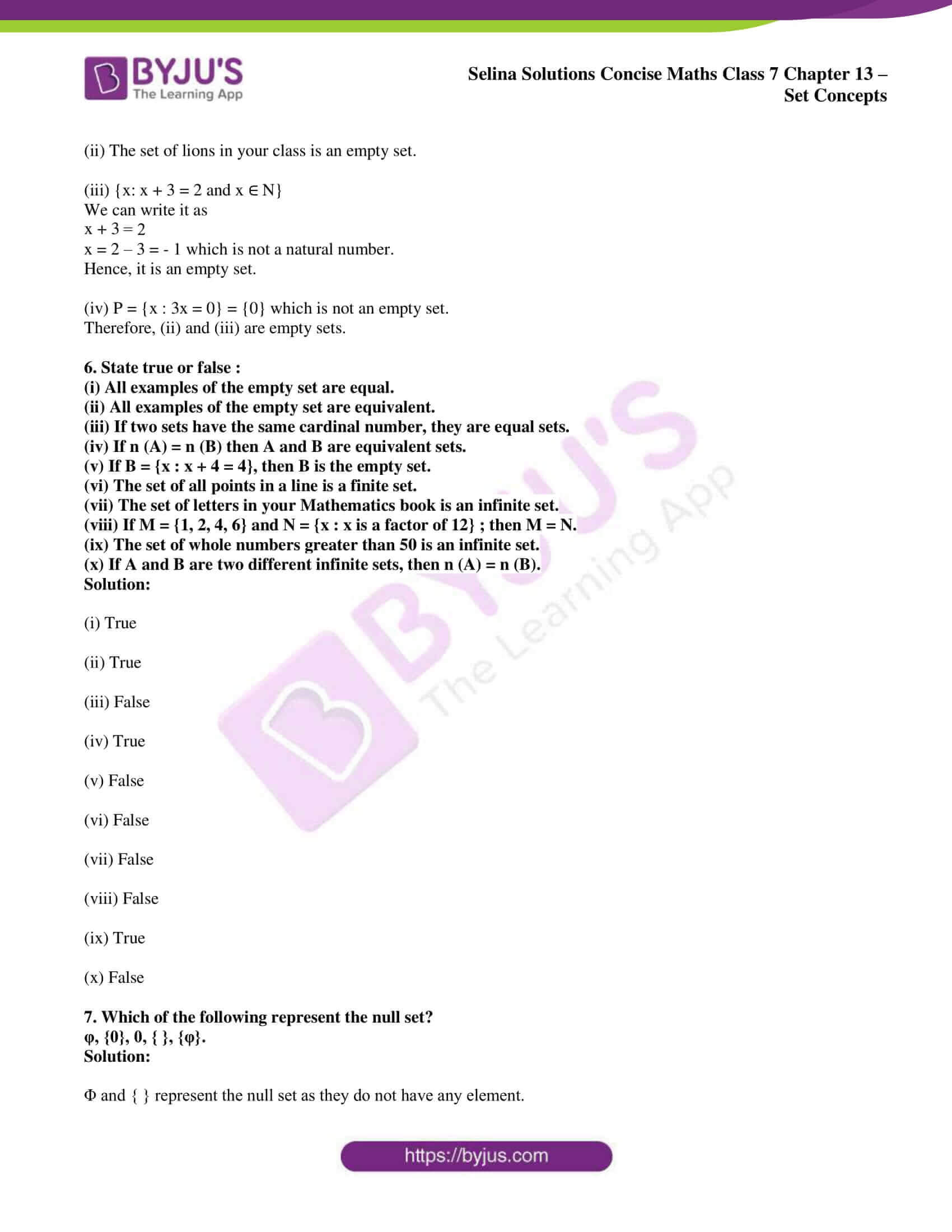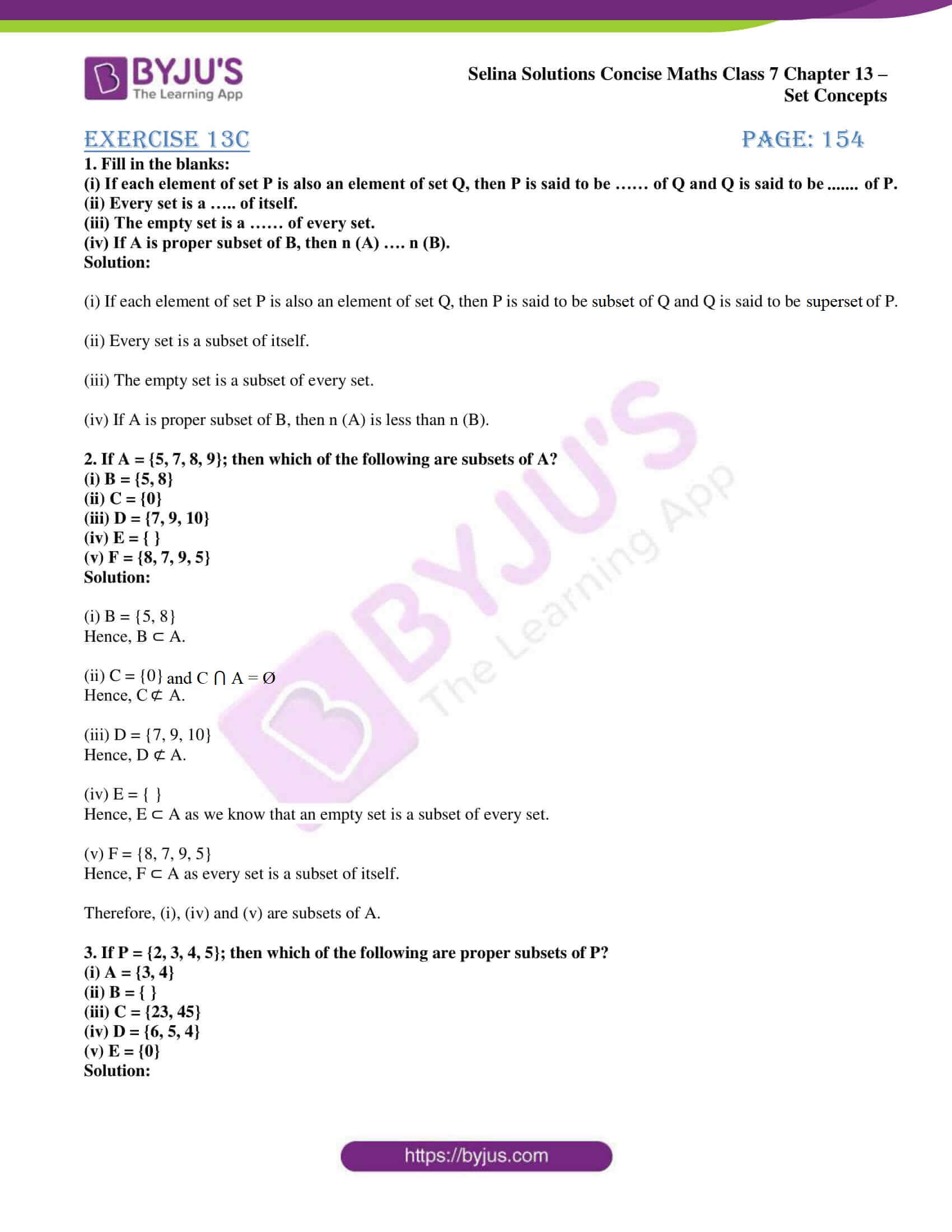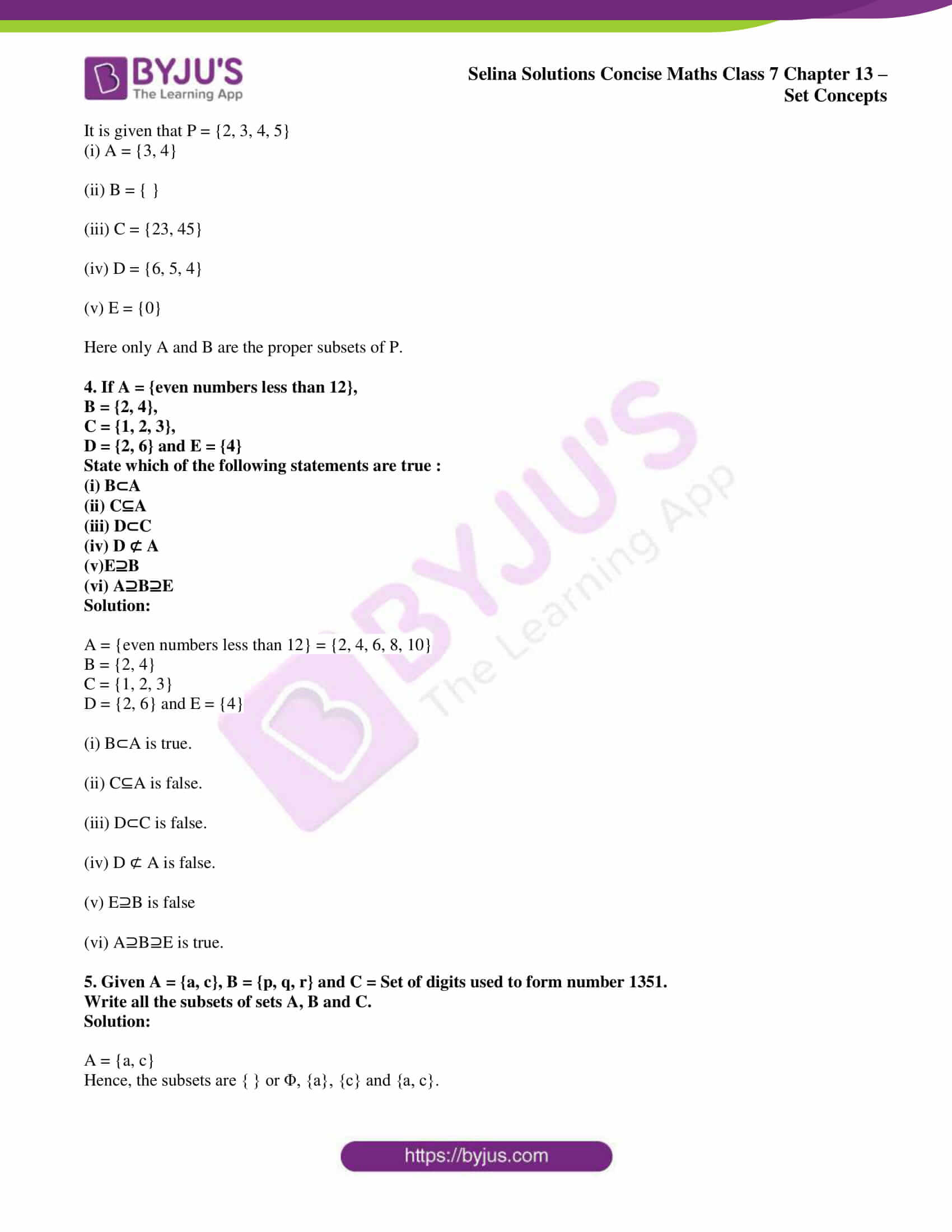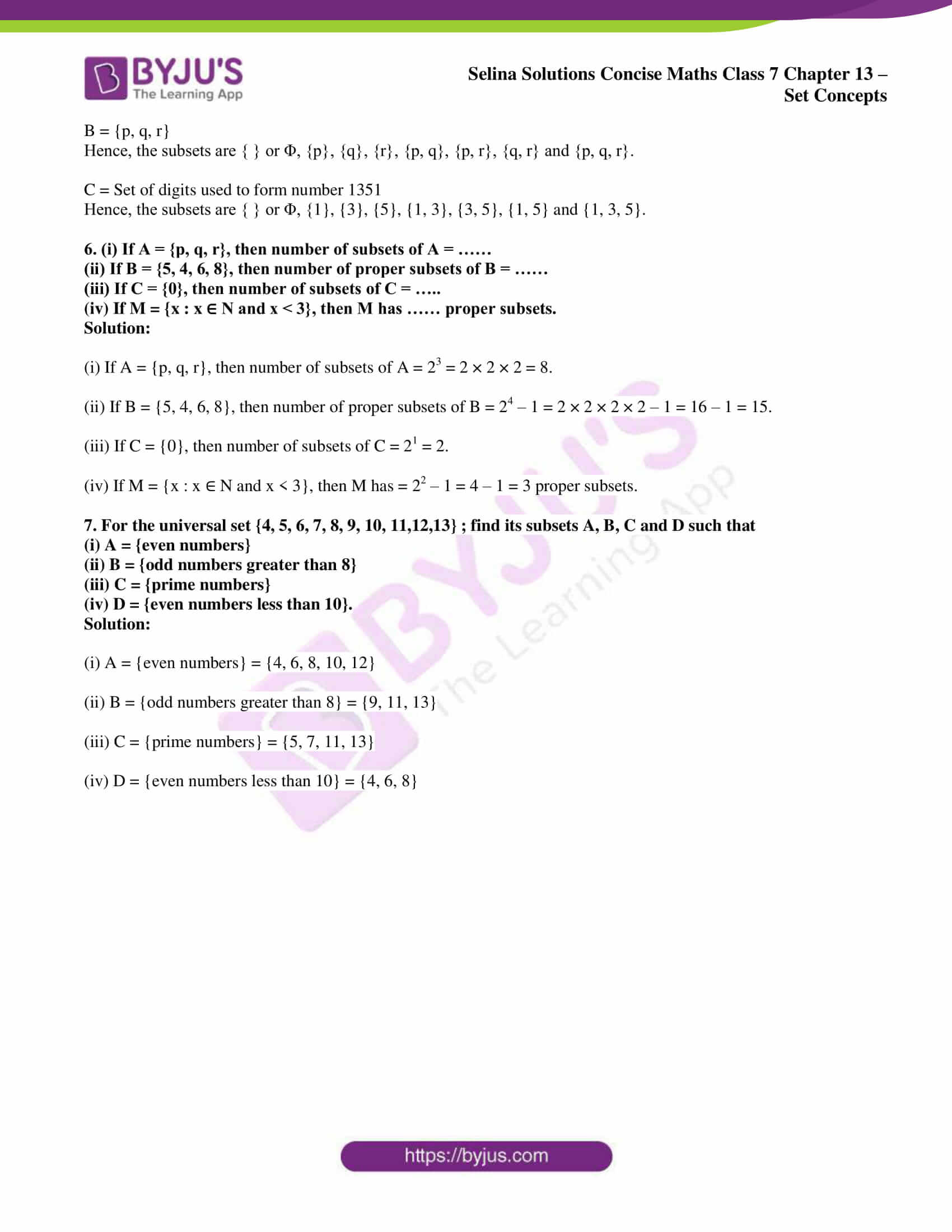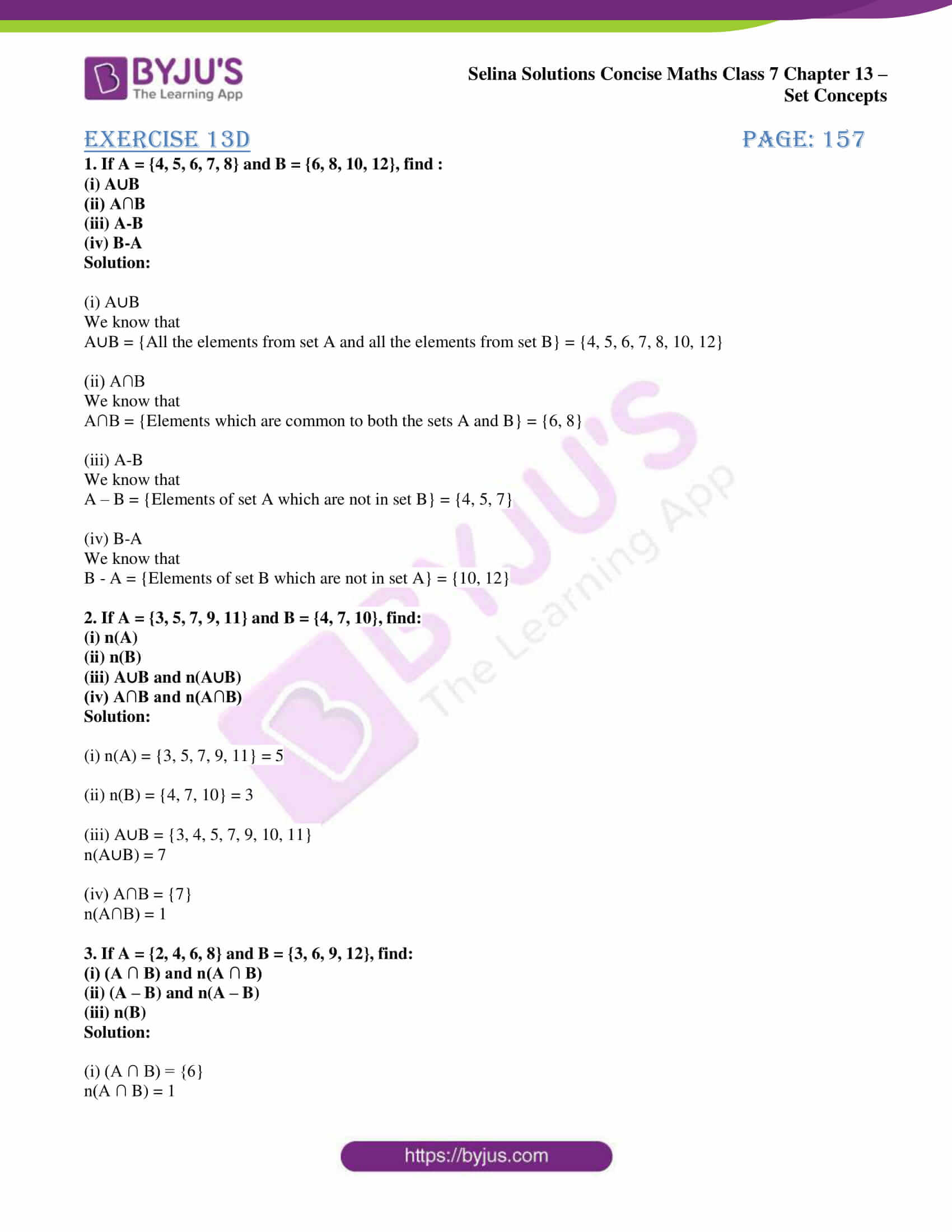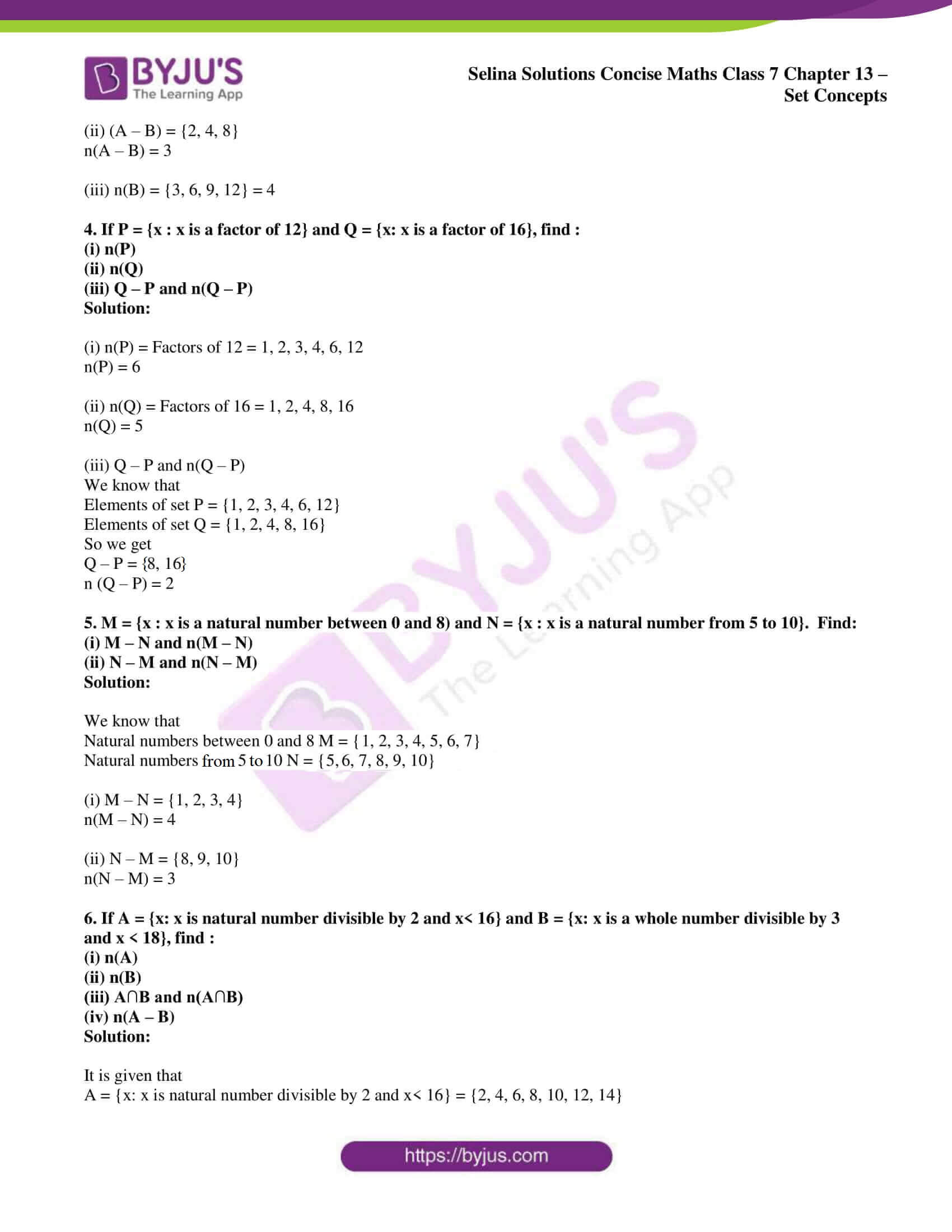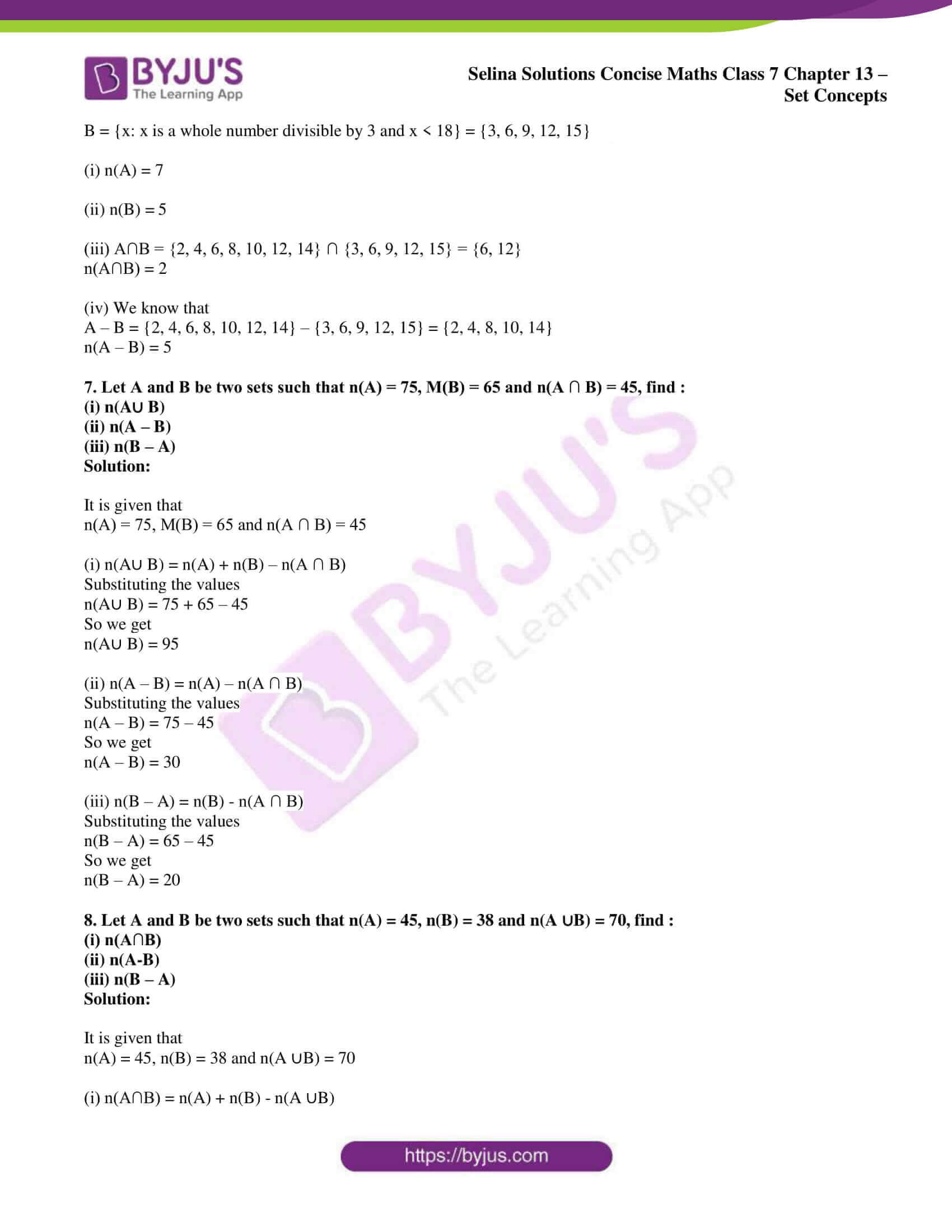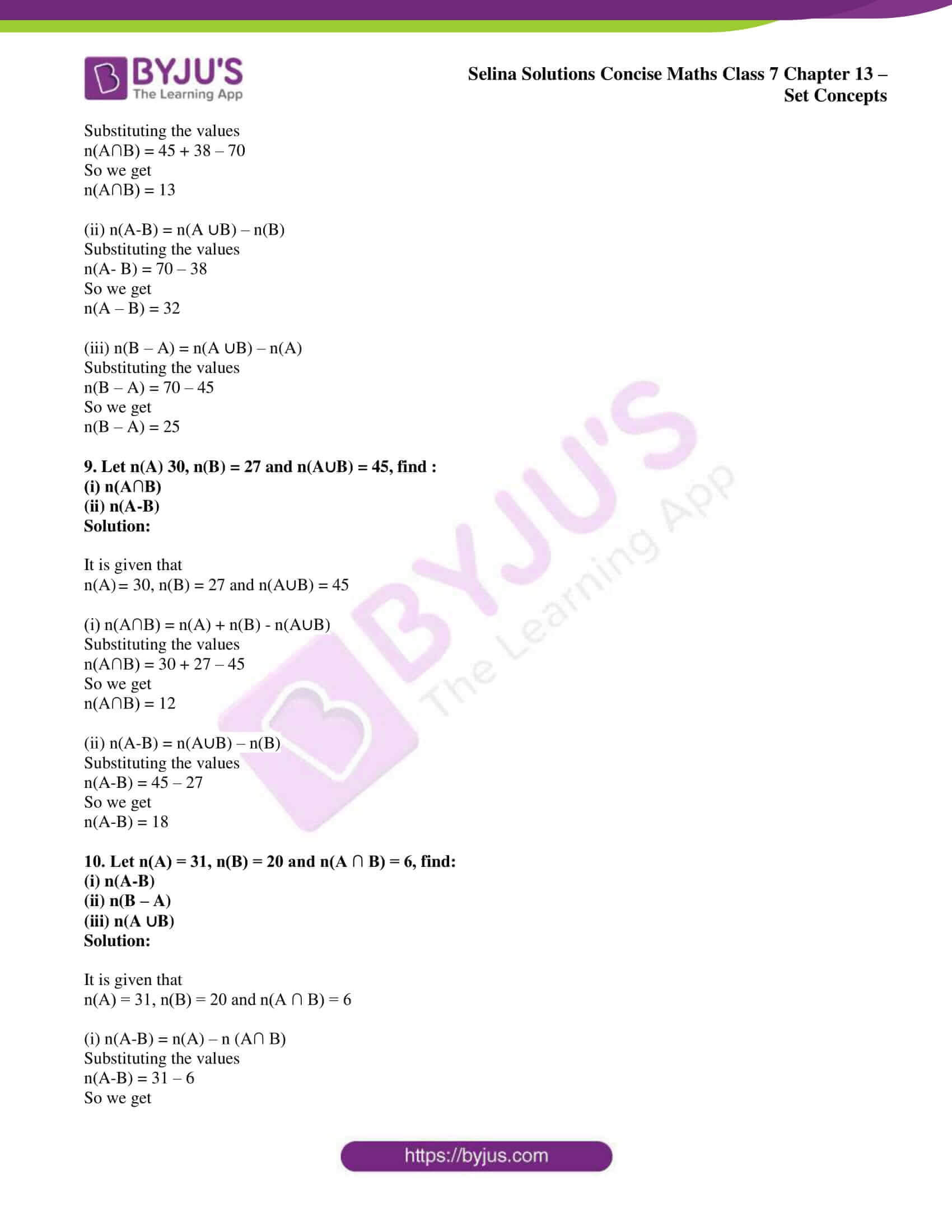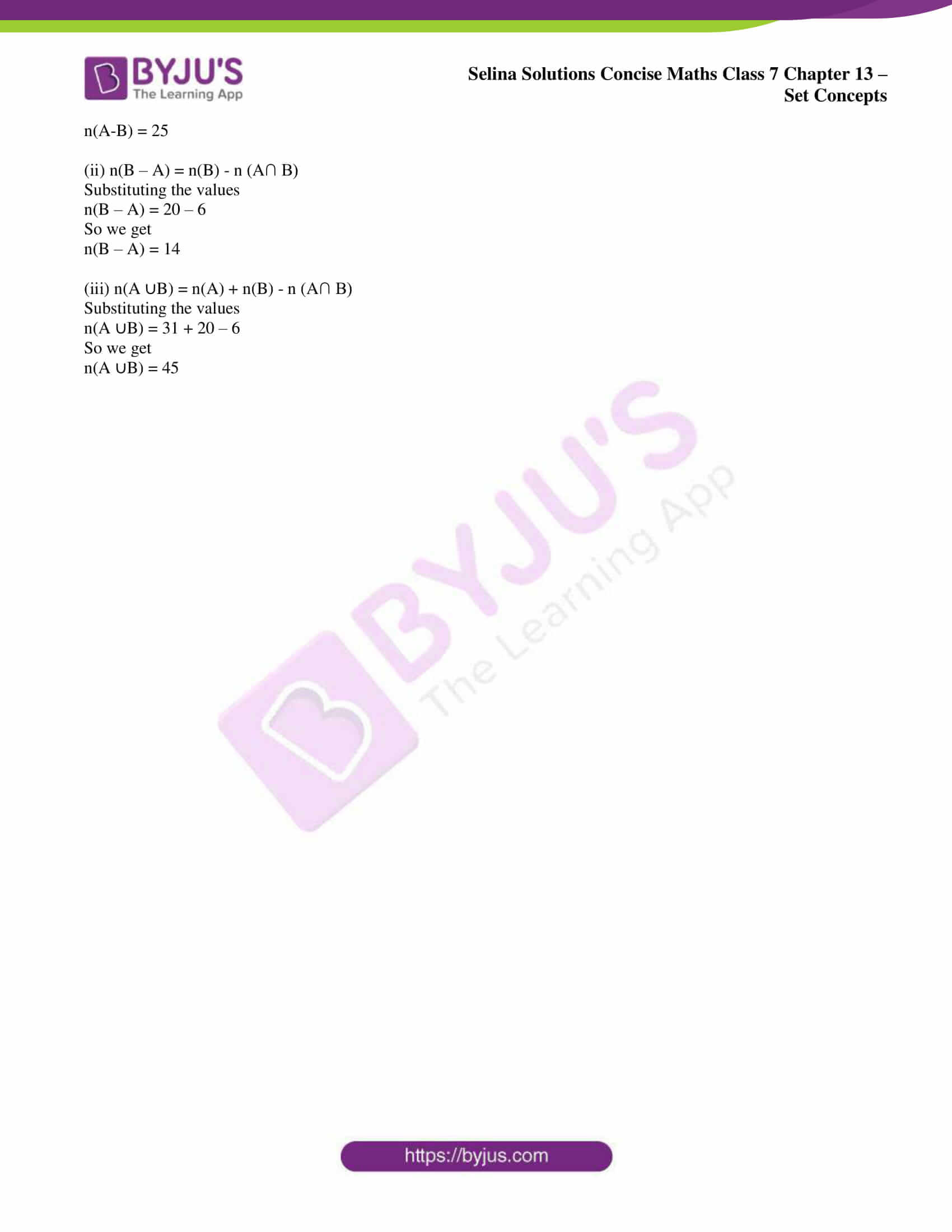### Exercises of Selina Solutions Concise Maths Class 7 Chapter 13 – Set Concepts

Exercise 13A Solutions

Exercise 13B Solutions

Exercise 13C Solutions

Exercise 13D Solutions

## Access Selina Solutions Concise Maths Class 7 Chapter 13: Set Concepts

#### Exercise 13A page: 148

1. Find, whether or not, each of the following collections represent a set:

(i) The collection of good students in your school.

(ii) The collection of the numbers between 30 and 45.

(iii) The collection of fat-people in your colony.

(iv) The collection of interesting books in your school library.

(v) The collection of books in the library and are of your interest.

Solution:

(i) The collection of good students in your school is not a set as it is not well defined.

(ii) The collection of the numbers between 30 and 45 is a set.

(iii) The collection of fat-people in your colony is not a set as it is not well defined.

(iv) The collection of interesting books in your school library is not a set as it is not well defined.

(v) The collection of books in the library and are of your interest is a set.

2. State whether true or false:

(i) Set {4, 5, 8} is same as the set {5, 4, 8} and the set {8, 4, 5}
(ii) Sets {a, b, m, n} and {a, a, m, b, n, n) are same.

(iii) Set of letters in the word ‘suchismita’ is {s, u, c, h, i, m, t, a}
(iv) Set of letters in the word ‘MAHMOOD’ is {M, A, H, O, D}.

Solution:

(i) It is true.

(ii) It is true.

(iii) It is true as {s, u, c, h, i, s, m, i, t, a} = {s, u, c, h, i, m, t, a}

(iv) It is true as it has the same elements.

3. Let set A = {6, 8, 10, 12} and set B = {3, 9, 15, 18}.
Insert the symbol ‘∈ ’ or ‘ ∉ ’ to make each of the following true :
(i) 6 …. A
(ii) 10 …. B
(iii) 18 …. B
(iv) (6 + 3) …. B
(v) (15 – 9) …. B
(vi) 12 …. A
(vii) (6 + 8) …. A
(viii) 6 and 8 …. A

Solution:

(i) 6 ∈ A

(ii) 10 ∉ B

(iii) 18 ∈ B

(iv) (6 + 3) or 9 ∈ B

(v) (15 – 9) or 6 ∉ B

(vi) 12 ∈ A

(vii) (6 + 8) or 14 ∉ A

(viii) 6 and 8 ∈ A

4. Express each of the following sets in
roster form :
(i) Set of odd whole numbers between 15 and 27.
(ii) A = Set of letters in the word “CHITAMBARAM”
(iii) B = {All even numbers from 15 to 26}
(iv) P = {x : x is a vowel used in the word ‘ARITHMETIC’}
(v) S = {Squares of first eight whole numbers}
(vi) Set of all integers between 7 and 94; which are divisible by 6.
(vii) C = {All composite numbers between 2 and 20}
(viii) D = Set of Prime numbers from 2 to 23.
(ix) E = Set of natural numbers below 30 which are divisible by 2 or 5.
(x) F = Set of factors of 24.
(xi) G = Set of names of three closed figures in Geometry.
(xii) H = {x : x
W and x < 10}
(xiii) J = {x: x
N and 2x – 3 ≤ 17}
(xiv) K = {x : x is an integer and – 3 < x < 5}

Solution:

(i) {17, 19, 21, 23, 25}

(ii) A = {C, H, I, T, A, M, B, R}

(iii) B = {16, 18, 20, 22, 24, 26}

(iv) P = {a, e, i}

(v) S = {0, 1, 4, 9, 16, 25, 36, 49}

(vi) {12, 18, 24, 30, 36, 42, 48, 54, 60, 66, 72, 78, 84, 90}

(vii) C = {4, 6, 8, 9, 10, 12, 14, 15, 16, 18}

(viii) D = {2, 3, 5, 7, 11, 13, 17, 19, 23}

(ix) E = {2, 4, 5, 6, 8, 10, 12, 14, 15, 16, 18, 20, 22, 24, 25, 26, 28}

(x) F = {1, 2, 3, 4, 6, 8, 12, 24}

(xi) G = {Triangle, Circle, Square}

(xii) H = {0, 1, 2, 3, 4, 5, 6, 7, 8, 9}

(xiii) 2x – 3 ≤ 17

By further calculation

2x ≤ 17 + 3

2x ≤ 20

So we get

x ≤ 20/2

x ≤ 10

Here J = {1, 2, 3, 4, 5, 6, 7, 8, 9, 10}

(xiv) -3 < x < 5

We know that x lies between – 3 and 5

So we get

K = {- 2, – 1, 0, 1, 2, 3, 4}

5. Express each of the following sets in set- builder notation (form):
(i) {3, 6, 9, 12, 15}
(ii) {2, 3, 5, 7, 11, 13 …. }
(iii) {1, 4, 9, 16, 25, 36}
(iv) {0, 2, 4, 6, 8, 10, 12, …. }
(v) {Monday, Tuesday, Wednesday}
(vi) {23, 25, 27, 29, … }

(vii) {1/3, ¼, 1/5, 1/6, 1/7, 1/8}

(viii) {42, 49, 56, 63, 70, 77}

Solution:

(i) {3, 6, 9, 12, 15}

It can be written as

= {x: x is a natural number divisible by 3; x < 18}

(ii) {2, 3, 5, 7, 11, 13 ….}

It can be written as

= {x: x is a prime number}

(iii) {1, 4, 9, 16, 25, 36}

It can be written as

= {x: x is a perfect square natural number; x ≤ 36}

(iv) {0, 2, 4, 6, 8, 10, 12, ….}

It can be written as

= {x: x is a whole number divisible by 2}

(v) {Monday, Tuesday, Wednesday}

It can be written as

= {x: x is one of the first three days of the week}

(vi) {23, 25, 27, 29, …}

It can be written as

= {x: x is an odd natural number; x ≥ 23}

(vii) {1/3, ¼, 1/5, 1/6, 1/7, 1/8}

It can be written as

= {x: x = 1/n when n is a natural number; 3 ≤ n ≤ 8}

(viii) {42, 49, 56, 63, 70, 77}

It can be written as

= {x: x is a natural number divisible by 7; 42 ≤ x ≤ 77}

6. Given: A = {x : x is a multiple of 2 and is less than 25}
B = {x : x is a square of a natural number and is less than 25}
C = {x : x is a multiple of 3 and is less than 25}
D = {x: x is a prime number less than 25}
Write the sets A, B, C and D in roster form.

Solution:

A = {x : x is a multiple of 2 and is less than 25} = {2, 4, 6, 8, 10, 12, 14, 16, 18, 20, 22, 24}

B = {x : x is a square of a natural number and is less than 25} = {1, 4, 9, 16}

C = {x : x is a multiple of 3 and is less than 25} = {3, 6, 9, 12, 15, 18, 21, 24}

D = {x: x is a prime number less than 25} = {2, 3, 5, 7, 11, 13, 17, 19, 23}

#### Exercise 13B page: 150

1. Write the cardinal number of each of the following sets:
(i) A = Set of days in a leap year.
(ii) B = Set of numbers on a clock-face.
(iii) C = {x : x ∈ N and x ≤ 7}
(iv) D = Set of letters in the word “PANIPAT”.
(v) E = Set of prime numbers between 5 and 15.
(vi) F = {x : x ∈ Z and – 2 < x ≤ 5}
(vii) G = {x : x is a perfect square number, x ∈ N and x ≤ 30}.

Solution:

(i) n (A) = 366

(ii) n (B) = 12

(iii) n (C) = 7

(iv) n (D) = 5

(v) n (E) = 3

(vi) n (F) = 7

(vii) n (G) = 5

2. For each set, given below, state whether it is finite set, infinite set or the null set :
(i) {natural numbers more than 100}
(ii) A = {x : x is an integer between 1 and 2}
(iii) B = {x : x ∈ W ; x is less than 100}.
(iv) Set of mountains in the world.
(v) {multiples of 8}.
(vi) {even numbers not divisible by 2}.
(vii) {squares of natural numbers}.
(viii) {coins used in India}
(ix) C = {x | x is a prime number between 7 and 10}.
(x) Planets of the Solar system.

Solution:

(i) {natural numbers more than 100}

It is an infinite set.

(ii) A = {x : x is an integer between 1 and 2}

It is a null set.

(iii) B = {x : x ∈ W ; x is less than 100}

It is a finite set as it contains 100 elements from 0 to 99.

(iv) Set of mountains in the world

It is an infinite set.

(v) {multiples of 8}

It is an infinite set.

(vi) {even numbers not divisible by 2}

It is a null set.

(vii) {squares of natural numbers}

It is an infinite set.

(viii) {coins used in India}

It is a finite set as it is countable.

(ix) C = {x | x is a prime number between 7 and 10}

There is no prime number between 7 to 10. It is a null set.

(x) Planets of the Solar system

It is a finite set as it is countable.

3. State, which of the following pairs of sets are disjoint :
(i) {0, 1, 2, 6, 8} and {odd numbers less than 10}.
(ii) {birds} and {trees}
(iii) {x : x is a fan of cricket} and {x : x is a fan of football}.
(iv) A = {natural numbers less than 10} and B = {x : x is a multiple of 5}.
(v) {people living in Calcutta} and {people living in West Bengal}.

Solution:

(i) {0, 1, 2, 6, 8} and {odd numbers less than 10}.

We can write it as

{0, 1, 2, 6, 8} and {1, 3, 5, 7, 9}

These are not disjoint sets as there is one element common.

(ii) {birds} and {trees}

These are disjoint sets as there is no common element.

(iii) {x : x is a fan of cricket} and {x : x is a fan of football}.

These are not disjoint sets as there can a person who is a fan of cricket and football.

(iv) A = {natural numbers less than 10} and B = {x : x is a multiple of 5}.

We can write it as

A = {1, 2, 3, 4, 5, 6, 7, 8, 9} and B = {5, 10, 15}

These are not disjoint sets as there is one element common.

(v) {people living in Calcutta} and {people living in West Bengal}.

These are not disjoint sets as Calcutta is a city of West Bengal.

4. State whether the given pairs of sets are equal or equivalent.
(i) A = {first four natural numbers} and B = {first four whole numbers}.
(ii) A = Set of letters of the word “FOLLOW” and B = Set of letters of the word “WOLF”.
(iii) E = {even natural numbers less than 10} and O = {odd natural numbers less than 9}
(iv) A = {days of the week starting with letter S} and B = {days of the week starting with letter T}.
(v) M = {multiples of 2 and 3 between 10 and 20} and N = {multiples of 2 and 5 between 10 and 20}.
(vi) P = {prime numbers which divide 70 exactly} and Q = {prime numbers which divide 105 exactly}
(vii) A = {0², 1², 2², 3², 4²} and B = {16, 9,4, 1, 0}.
(viii) E = {8, 1O, 12, 14, 16} and F = {even natural numbers between 6 and 18}.
(ix) A = {letters of the word SUPERSTITION} and B = {letters of the word JURISDICTION}.

Solution:

(i) A = {first four natural numbers} = {1, 2, 3, 4}

B = {first four whole numbers} = {0, 1, 2, 3}

It is an equivalent set as both have equal number of elements which are not same.

(ii) A = Set of letters of the word “FOLLOW” = {F, 0, L, W}

B = Set of letters of the word “WOLF” = {W, O, L, F}

It is an equal set as both have same and equal elements.

(iii) E = {even natural numbers less than 10} = {2, 4, 6, 8}

O = {odd natural numbers less than 9} = {1, 3, 5, 7}

It is an equivalent set as both have equal number of elements which are not same.

(iv) A = {days of the week starting with letter S} = {Sunday, Saturday}

B = {days of the week starting with letter T} = {Tuesday, Thursday}

It is an equivalent set as both have equal number of elements which are not same.

(v) M = {multiples of 2 and 3 between 10 and 20} = {12, 14, 15, 16, 18}

N = {multiples of 2 and 5 between 10 and 20} = {12, 14, 15, 16, 18}

It is an equal set as both have same and equal elements.

(vi) P = {prime numbers which divide 70 exactly} = {2, 5, 7}

Q = {prime numbers which divide 105 exactly} = {3, 5, 7}

It is an equivalent set as both have equal number of elements which are not same.

(vii) A = {0², 1², 2², 3², 4²} = {0, 1, 4, 9, 16}

B = {16, 9, 4, 1, 0}

It is an equal set as both have same and equal elements.

(viii) E = {8, 10, 12, 14, 16}

F = {even natural numbers between 6 and 18} = {8, 10, 12, 14, 16}

It is an equal set as both have same and equal elements.

(ix) A = {letters of the word SUPERSTITION} = {S, U, P, E, R, T, I, O, N}

B = {letters of the word JURISDICTION} = {J, U, R, I, S, D, C, T, O, N}

It is neither equal nor equivalent sets as they have different and unequal elements.

5. Examine which of the following sets are the empty sets :
(i) The set of triangles having three equal sides.
(ii) The set of lions in your class.
(iii) {x: x + 3 = 2 and x ∈ N}
(iv) P = {x : 3x = 0}

Solution:

(i) The set of triangles having three equal sides is not an empty set.

(ii) The set of lions in your class is an empty set.

(iii) {x: x + 3 = 2 and x ∈ N}

We can write it as

x + 3 = 2

x = 2 – 3 = – 1 which is not a natural number.

Hence, it is an empty set.

(iv) P = {x : 3x = 0} = {0} which is not an empty set.

Therefore, (ii) and (iii) are empty sets.

6. State true or false :
(i) All examples of the empty set are equal.
(ii) All examples of the empty set are equivalent.
(iii) If two sets have the same cardinal number, they are equal sets.
(iv) If n (A) = n (B) then A and B are equivalent sets.
(v) If B = {x : x + 4 = 4}, then B is the empty set.
(vi) The set of all points in a line is a finite set.
(vii) The set of letters in your Mathematics book is an infinite set.
(viii) If M = {1, 2, 4, 6} and N = {x : x is a factor of 12} ; then M = N.
(ix) The set of whole numbers greater than 50 is an infinite set.
(x) If A and B are two different infinite sets, then n (A) = n (B).

Solution:

(i) True

(ii) True

(iii) False

(iv) True

(v) False

(vi) False

(vii) False

(viii) False

(ix) True

(x) False

7. Which of the following represent the null set?
φ, {0}, 0, { }, {φ}.

Solution:

Φ and { } represent the null set as they do not have any element.

#### Exercise 13C page: 154

1. Fill in the blanks:

(i) If each element of set P is also an element of set Q, then P is said to be …… of Q and Q is said to be …… of P.
(ii) Every set is a ….. of itself.
(iii) The empty set is a …… of every set.
(iv) If A is proper subset of B, then n (A) …. n (B).

Solution:

(i) If each element of set P is also an element of set Q, then P is said to be a subset of Q and Q is said to be the superset of P.

(ii) Every set is a subset of itself.

(iii) The empty set is a subset of every set.

(iv) If A is proper subset of B, then n (A) is less than n (B).

2. If A = {5, 7, 8, 9}; then which of the following are subsets of A?
(i) B = {5, 8}
(ii) C = {0}
(iii) D = {7, 9, 10}
(iv) E = { }
(v) F = {8, 7, 9, 5}

Solution:

(i) B = {5, 8}

Hence, B ⊂ A.

(ii) C = {0} and C  ⋂ A = Ø

Hence, C ⊄ A.

(iii) D = {7, 9, 10}

Hence, D ⊄ A.

(iv) E = { }

Hence, E ⊂ A as we know that an empty set is a subset of every set.

(v) F = {8, 7, 9, 5}

Hence, F ⊂ A as every set is a subset of itself.

Therefore, (i), (iv) and (v) are subsets of A.

3. If P = {2, 3, 4, 5}; then which of the following are proper subsets of P?
(i) A = {3, 4}
(ii) B = { }
(iii) C = {23, 45}
(iv) D = {6, 5, 4}
(v) E = {0}

Solution:

It is given that P = {2, 3, 4, 5}

(i) A = {3, 4}

(ii) B = { }

(iii) C = {23, 45}

(iv) D = {6, 5, 4}

(v) E = {0}

Here only A and B are the proper subsets of P.

4. If A = {even numbers less than 12},
B = {2, 4},
C = {1, 2, 3},
D = {2, 6} and E = {4}
State which of the following statements are true :
(i) B⊂A
(ii) C⊆A
(iii) D⊂C
(iv) D ⊄ A
(v)E⊇B
(vi) A⊇B⊇E

Solution:

A = {even numbers less than 12} = {2, 4, 6, 8, 10}

B = {2, 4}

C = {1, 2, 3}

D = {2, 6} and E = {4}

(i) B⊂A is true.

(ii) C⊆A is false.

(iii) D⊂C is false.

(iv) D ⊄ A is false.

(v) E⊇B is false

(vi) A⊇B⊇E is true.

5. Given A = {a, c}, B = {p, q, r} and C = Set of digits used to form number 1351.
Write all the subsets of sets A, B and C.

Solution:

A = {a, c}

Hence, the subsets are { } or Φ, {a}, {c} and {a, c}.

B = {p, q, r}

Hence, the subsets are { } or Φ, {p}, {q}, {r}, {p, q}, {p, r}, {q, r} and {p, q, r}.

C = Set of digits used to form number 1351

Hence, the subsets are { } or Φ, {1}, {3}, {5}, {1, 3}, {3, 5}, {1, 5} and {1, 3, 5}.

6. (i) If A = {p, q, r}, then number of subsets of A = ……
(ii) If B = {5, 4, 6, 8}, then number of proper subsets of B = ……
(iii) If C = {0}, then number of subsets of C = …..
(iv) If M = {x : x ∈ N and x < 3}, then M has …… proper subsets.

Solution:

(i) If A = {p, q, r}, then number of subsets of A = 23 = 2 × 2 × 2 = 8.

(ii) If B = {5, 4, 6, 8}, then number of proper subsets of B = 24 – 1 = 2 × 2 × 2 × 2 – 1 = 16 – 1 = 15.

(iii) If C = {0}, then number of subsets of C = 21 = 2.

(iv) If M = {x : x ∈ N and x < 3}, then M has = 22 – 1 = 4 – 1 = 3 proper subsets.

7. For the universal set {4, 5, 6, 7, 8, 9, 10, 11,12,13} ; find its subsets A, B, C and D such that
(i) A = {even numbers}
(ii) B = {odd numbers greater than 8}
(iii) C = {prime numbers}
(iv) D = {even numbers less than 10}.
Solution:

(i) A = {even numbers} = {4, 6, 8, 10, 12}

(ii) B = {odd numbers greater than 8} = {9, 11, 13}

(iii) C = {prime numbers} = {5, 7, 11, 13}

(iv) D = {even numbers less than 10} = {4, 6, 8}

#### Exercise 13D page: 157

1. If A = {4, 5, 6, 7, 8} and B = {6, 8, 10, 12}, find :
(i) A∪B
(ii) A∩B
(iii) A-B
(iv) B-A

Solution:

(i) A∪B

We know that

A∪B = {All the elements from set A and all the elements from set B} = {4, 5, 6, 7, 8, 10, 12}

(ii) A∩B

We know that

A∩B = {Elements which are common to both the sets A and B} = {6, 8}

(iii) A-B

We know that

A – B = {Elements of set A which are not in set B} = {4, 5, 7}

(iv) B-A

We know that

B – A = {Elements of set B which are not in set A} = {10, 12}

2. If A = {3, 5, 7, 9, 11} and B = {4, 7, 10}, find:
(i) n(A)
(ii) n(B)
(iii) A∪B and n(A∪B)
(iv) A∩B and n(A∩B)

Solution:

(i) n(A) = {3, 5, 7, 9, 11} = 5

(ii) n(B) = {4, 7, 10} = 3

(iii) A∪B = {3, 4, 5, 7, 9, 10, 11}

n(A∪B) = 7

(iv) A∩B = {7}

n(A∩B) = 1

3. If A = {2, 4, 6, 8} and B = {3, 6, 9, 12}, find:
(i) (A ∩ B) and n(A ∩ B)
(ii) (A – B) and n(A – B)
(iii) n(B)

Solution:

(i) (A ∩ B) = {6}

n(A ∩ B) = 1

(ii) (A – B) = {2, 4, 8}

n(A – B) = 3

(iii) n(B) = {3, 6, 9, 12} = 4

4. If P = {x : x is a factor of 12} and Q = {x: x is a factor of 16}, find :
(i) n(P)
(ii) n(Q)
(iii) Q – P and n(Q – P)

Solution:

(i) n(P) = Factors of 12 = 1, 2, 3, 4, 6, 12

n(P) = 6

(ii) n(Q) = Factors of 16 = 1, 2, 4, 8, 16

n(Q) = 5

(iii) Q – P and n(Q – P)

We know that

Elements of set P = {1, 2, 3, 4, 6, 12}

Elements of set Q = {1, 2, 4, 8, 16}

So we get

Q – P = {8, 16}

n (Q – P) = 2

5. M = {x : x is a natural number between 0 and 8) and N = {x : x is a natural number from 5 to 10}. Find:
(i) M – N and n(M – N)
(ii) N – M and n(N – M)

Solution:

We know that

Natural numbers between 0 and 8 M = {1, 2, 3, 4, 5, 6, 7}

Natural numbers from 5 to 10 N = {5, 6, 7, 8, 9, 10}

(i) M – N = {1, 2, 3, 4}

n(M – N) = 4

(ii) N – M = {8, 9, 10}

n(N – M) = 3

6. If A = {x: x is natural number divisible by 2 and x< 16} and B = {x: x is a whole number divisible by 3 and x < 18}, find :
(i) n(A)
(ii) n(B)
(iii) A∩B and n(A∩B)
(iv) n(A – B)

Solution:

It is given that

A = {x: x is natural number divisible by 2 and x< 16} = {2, 4, 6, 8, 10, 12, 14}

B = {x: x is a whole number divisible by 3 and x < 18} = {3, 6, 9, 12, 15}

(i) n(A) = 7

(ii) n(B) = 5

(iii) A∩B = {2, 4, 6, 8, 10, 12, 14} ∩ {3, 6, 9, 12, 15} = {6, 12}

n(A∩B) = 2

(iv) We know that

A – B = {2, 4, 6, 8, 10, 12, 14} – {3, 6, 9, 12, 15} = {2, 4, 8, 10, 14}

n(A – B) = 5

7. Let A and B be two sets such that n(A) = 75, M(B) = 65 and n(A ∩ B) = 45, find :
(i) n(A∪ B)
(ii) n(A – B)
(iii) n(B – A)

Solution:

It is given that

n(A) = 75, M(B) = 65 and n(A ∩ B) = 45

(i) n(A∪ B) = n(A) + n(B) – n(A ∩ B)

Substituting the values

n(A∪ B) = 75 + 65 – 45

So we get

n(A∪ B) = 95

(ii) n(A – B) = n(A) – n(A ∩ B)

Substituting the values

n(A – B) = 75 – 45

So we get

n(A – B) = 30

(iii) n(B – A) = n(B) – n(A ∩ B)

Substituting the values

n(B – A) = 65 – 45

So we get

n(B – A) = 20

8. Let A and B be two sets such that n(A) = 45, n(B) = 38 and n(A ∪B) = 70, find :
(i) n(A∩B)
(ii) n(A-B)
(iii) n(B – A)

Solution:

It is given that

n(A) = 45, n(B) = 38 and n(A ∪B) = 70

(i) n(A∩B) = n(A) + n(B) – n(A ∪B)

Substituting the values

n(A∩B) = 45 + 38 – 70

So we get

n(A∩B) = 13

(ii) n(A-B) = n(A ∪B) – n(B)

Substituting the values

n(A- B) = 70 – 38

So we get

n(A – B) = 32

(iii) n(B – A) = n(A ∪B) – n(A)

Substituting the values

n(B – A) = 70 – 45

So we get

n(B – A) = 25

9. Let n(A) 30, n(B) = 27 and n(A∪B) = 45, find :
(i) n(A∩B)
(ii) n(A-B)

Solution:

It is given that

n(A) = 30, n(B) = 27 and n(A∪B) = 45

(i) n(A∩B) = n(A) + n(B) – n(A∪B)

Substituting the values

n(A∩B) = 30 + 27 – 45

So we get

n(A∩B) = 12

(ii) n(A-B) = n(A∪B) – n(B)

Substituting the values

n(A-B) = 45 – 27

So we get

n(A-B) = 18

10. Let n(A) = 31, n(B) = 20 and n(A ∩ B) = 6, find:
(i) n(A-B)
(ii) n(B – A)
(iii) n(A ∪B)

Solution:

It is given that

n(A) = 31, n(B) = 20 and n(A ∩ B) = 6

(i) n(A-B) = n(A) – n (A∩ B)

Substituting the values

n(A-B) = 31 – 6

So we get

n(A-B) = 25

(ii) n(B – A) = n(B) – n (A∩ B)

Substituting the values

n(B – A) = 20 – 6

So we get

n(B – A) = 14

(iii) n(A ∪B) = n(A) + n(B) – n (A∩ B)

Substituting the values

n(A ∪B) = 31 + 20 – 6

So we get

n(A ∪B) = 45